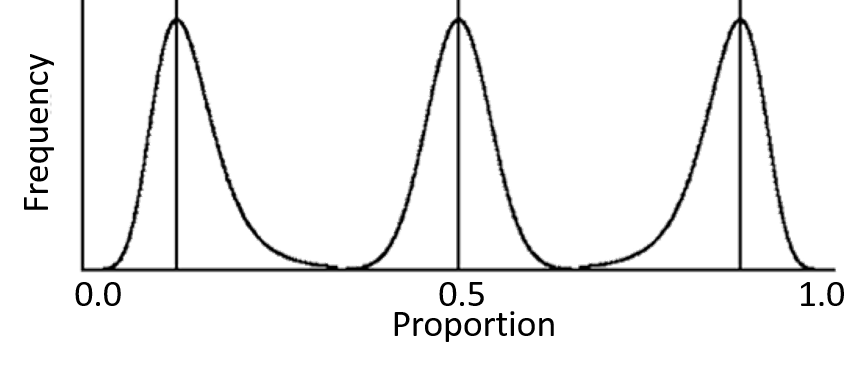Content DisclaimerCopyright @2020.All Rights Reserved.
StatsToDo : Sample Size for Estimating Population Proportions
 Introduction Javascript Program R Codes Tables This page presents methods for estimating sample size requirements to establish a proportion. This is frequently needed, to establish the frequencies of events, such as death rates, complication rates, success rates, and so on. The first approach is to assume that binomial distribution is approximately the same as normal distribution, with the Standard Error depending on the proportion and sample size. From this, the sample size required and the error resulted can be calculated according to the normal distribution model.It is also recognised that the binomial distribution is only similar to the normal distribution when the proportion is near 0.5, as the confidence intervals cannot overlap 0 or 1. This means that the distribution becomes increasingly skewed as the proportion becomes increasingly away from 0.5, and this skewness is pronounced when the sample size is small, as shown in the diagram to the left The second approach is therefore to accept that the binomial distribution is not the same as normal distribution, that is is skewed, narrower towars the ends (0 or 1) and wider towards the middle (0.5). When setting the parameters for calculations, the errors on the two sides of the proportion must be recognised and specified as lower (towards 0) or higher (towards 1). Terminology Confidence is the percent confidence, commonly 80%, 90%, 95%, or 99% Proportion (prop) is the proportion Error (er) is the error When normal distribution is assumed, the confidenc interval is proportion±error When binomial distribution is assumed, the confidence interval is from proportion - error(lower side) to proportion + error(higher side) Sample size is the sample size required to secure the tolerable error given a proportion When normal distribution is assumed, the sample size depends on the symmetrical error When binomial distribution is assumed, the sample size differs depending on whether the error refers to the lower or the higher side Choice of Method When the proportion is not near end extremes (say 0.15 to 0.85), and the sample size is large (say both the number of positive and negative cases exceed 20), results of calculation assuming normal or binomial distribution are similar and the error on on both side are symmetrical. When the proportion concerned is nearer the extremes (<0.15 or >0.85) and the samle size is likely to be small, the more robust binomial distribution should be used References Machin D, Campbell M, Fayers, P, Pinol A (1997) Sample Size Tables for Clinical Studies. Second Ed. Blackwell Science IBSN 0-86542-870-0 p. 135